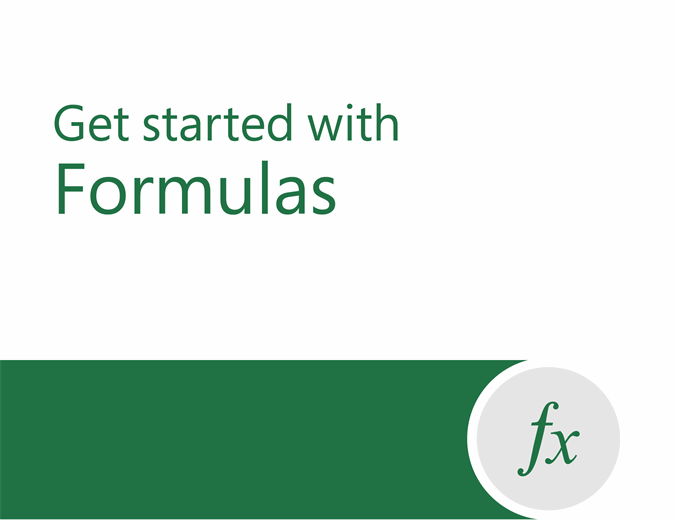Simplify the complex with data types in Excel. DOWNLOAD THE TEMPLATES NOW.

# Formula tutorial

If you’re new to Excel, or even if you have some experience with it, you can walk through Excel’s most common formulas in this Excel formula tutorial template. With real-world examples and helpful visuals, you’ll be able to Sum, Count, Average, and VLOOKUP like a pro with the help of this Excel formula tutorial template.

Excel